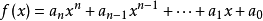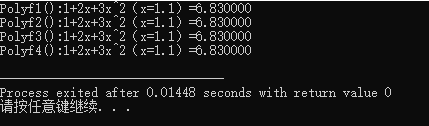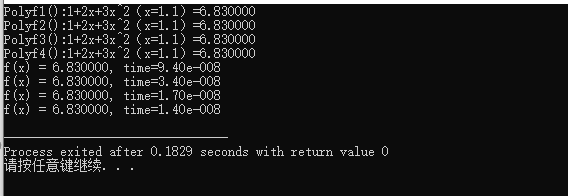• C语言求多项式求sinx近似。来源:互联网宽屏版评论2010-12-22 19:59:48分类: 电脑/网络 >> 程序设计 >> 其他编程语言问题描述:3、利用下列台劳多项式求sinx近似。Sin x ≈x/1 - x3/3! + x5/5!-...
用C语言求多项式求sinx的近似值。来源:互联网  宽屏版  评论2010-12-22 19:59:48分类: 电脑/网络 >> 程序设计 >> 其他编程语言问题描述:3、利用下列台劳多项式求sinx的近似值。Sin x ≈x/1 - x3/3! + x5/5!-x7/7!+…….+(-1)n-1x2n-1 /(2n-1)!参考答案:#include double factorial(double n){//求n!double result=1;for(int i=1;i<=n;i++){result*=i;}return result;}double sinx(double x,int n){//求sin(x),精度为ndouble result=0;bool bAdd=true;//是加还是减for(int i=1;i<=n;i++){if(bAdd){result+=((double)x*(2*i-1)/factorial(2*i-1));}else{result-=((double)x*(2*i-1)/factorial(2*i-1));}bAdd=!bAdd;}return result;}void main(){printf("%f\r\n",sinx(0.4,10));}[b]分类:[/b] 电脑/网络 >> 程序设计 >> 其他编程语言[br][b]问题描述:[/b][br]3、利用下列台劳多项式求sinx的近似值。Sin x ≈x/1 - x3/3! + x5/5!-x7/7!+…….+(-1)n-1x2n-1 /(2n-1)![br][b]参考答案:[/b][br]#include double factorial(double n){//求n!double result=1;for(int i=1;i<=n;i++){result*=i;}return result;}double sinx(double x,int n){//求sin(x),精度为ndouble result=0;bool bAdd=true;//是加还是减for(int i=1;i<=n;i++){if(bAdd){result+=((double)x*(2*i-1)/factorial(2*i-1));}else{result-=((double)x*(2*i-1)/factorial(2*i-1));}bAdd=!bAdd;}return result;}void main(){printf("%f\r\n",sinx(0.4,10));}
展开全文• ## c语言-多项式求值

千次阅读 2019-03-06 20:50:39
6-2多项式值（15 分） 本题要求实现一个函数，计算阶数为n，系数为a...a[n]的多项式f(x)=∑​i=0​n...其中n是多项式的阶数，a[]中存储系数，x是给定点。函数须返回多项式f(x)的值。 裁判测试程序样例： #...
6-2 多项式求值 （15 分）

本题要求实现一个函数，计算阶数为n，系数为a ... a[n]的多项式f(x)=∑​i=0​n​​(a[i]×x​i​​) 在x点的值。

函数接口定义：

double f( int n, double a[], double x );


其中n是多项式的阶数，a[]中存储系数，x是给定点。函数须返回多项式f(x)的值。

裁判测试程序样例：

#include <stdio.h>

#define MAXN 10

double f( int n, double a[], double x );

int main()
{
int n, i;
double a[MAXN], x;

scanf("%d %lf", &n, &x);
for ( i=0; i<=n; i++ )
scanf(“%lf”, &a[i]);
printf("%.1f\n", f(n, a, x));
return 0;
}

/* 你的代码将被嵌在这里 */


输入样例：

2 1.1
1 2.5 -38.7


输出样例：

-43.1

程序：

#include <stdio.h>

#define MAXN 10

double f( int n, double a[], double x );

int main()
{
int n, i;
double a[MAXN], x;

scanf("%d %lf", &n, &x);
for ( i=0; i<=n; i++ )
scanf(“%lf”, &a[i]);
printf("%.1f\n", f(n, a, x));
return 0;
}
double f( int n, double a[], double x )
{
int i;
double b=1.0;
double sum=a;
for(i=1;i<=n;i++)
{
b=b*x;
sum=sum+b*a[i];
}
return sum;
}


展开全文• (1)表达式求值是程序设计语言编译中一个最基本问题。通常，表达式由运算符、操作数、界限符组成，算术表达式中最常见表示法形式有中缀、前缀和后缀表示法。中缀表示法是书写表达式常见方式，前缀和后缀表示...

戳这里还有其他数据结构的题目噢

https://blog.csdn.net/qq_45724947/article/details/115625130?spm=1001.2014.3001.5501

设计并验证以下算法:首先将一个中缀表达式转换成逆波兰式，然后对此逆波兰表达式求值。
(1)表达式求值是程序设计语言编译中的一个最基本的问题。通常，表达式由运算符、操作数、界限符组成，算术表达式中最常见的表示法形式有中缀、前缀和后缀表示法。中缀表示法是书写表达式的常见方式，前缀和后缀表示法主要用于计算机科学领域。
①中缀表达式:运算符在两操作数的中间。在运算中存在运算符的优先权与结合性的问题。例如运算a/b-(c+d)*f时，编译器即自左向右逐一检查，当检查到第一个运算符“/”时还无法知道是否执行；待检查到第二个运算符“-”时，因为知道“/”的优先级别高于“-”时，才知道执行“a/b"；当继续检查到“(”时，可知道先执行括号以内部分。
②前缀表达式:运算符放在两操作数的前面。这种表示法经常用于计算机科学，特别是编译器设计方面。为纪念其发明家Jan Lukasiewicz,该表示法也称波兰表示法。
③后缀表达式:运算符放在两操作数的后面。后缀表达式也称逆波兰表达式，因其使表达式求值变得轻松，所以被普遍使用。
前缀和后缀表示法有以下共同特征:操作数的顺序与等价的中缀表达式中操作数的顺序一致、不需要括号、操作符的优先级不相关。
(2)要求从键盘读入一个包括加减乘除、括号与正整数的中缀表达式，将其转换成后缀表达式存入一张线性表并输出，对后缀表达式求值并输出。
(3)将中缀表达式转换成后缀表达式的过程中，需要定义一个运算符栈；对后缀表达式求值的过程中，需要定义一个操作数栈。
(4)教材“表3.1 算符间的优先关系”可用一个二维字符数组来描述:
char Prior={‘>’,‘>’,‘<’,‘<’,‘<’,‘>’,‘>’,
‘>’,‘>’,‘<’,‘<’,‘<’,‘>’,‘>’,
‘>’,‘>’,‘>’,‘>’,‘<’,‘>’,‘>’,
‘>’,‘>’,‘>’,‘>’,‘<’,‘>’,‘>’,
‘<’,‘<’,‘<’,‘<’,‘<’,‘=’,‘ ’,
‘>’,‘>’,‘>’,‘>’,‘ ’,‘>’,‘>’,
‘<’,‘<’,‘<’,‘<’,‘<’,‘ ’,‘=’}

直接上代码：

#include<stdio.h>
#include<stdlib.h>
#include<assert.h>
#include<ctype.h>

#define INITSIZE  20
#define INCREMENT 10
#define MAX 20
#define LEN   sizeof(Elemtype)

/*栈的动态分配顺序存储结构*/
typedef char Elemtype;
typedef double elem;
typedef struct{
Elemtype *base;
Elemtype *top;
int StackSize;
}SqStack;
typedef struct{
elem *base;
elem *top;
int StackSize;
}Sq;

void InitStack(SqStack *S)//初始化 栈 111111
{
S->base=(Elemtype*)malloc(LEN*INITSIZE);
assert(S->base != NULL);
S->top = S->base;
S->StackSize = INITSIZE;
}

void PushStack(SqStack *S,Elemtype e)//压栈
{
if(S->top - S->base >= S->StackSize)
{
S->base=(Elemtype*)realloc(S->base,(S->StackSize+INCREMENT)*LEN);
assert(S->base != NULL);
S->top = S->base + S->StackSize ;
S->StackSize += INCREMENT;
}
*S->top = e;
S->top++;
}

void PopStack(SqStack *S,Elemtype *e)//弹栈
{
*e=*--S->top;
}

void Init(Sq *S)//初始化 栈 111111
{
S->base=(elem*)malloc(LEN*INITSIZE);
assert(S->base != NULL);
S->top = S->base;
S->StackSize = INITSIZE;
}

void Push(Sq *S,double e)//压栈
{
if(S->top - S->base >= S->StackSize)
{
S->base=(double*)realloc(S->base,(S->StackSize+INCREMENT)*LEN);
assert(S->base != NULL);
S->top = S->base + S->StackSize ;
S->StackSize += INCREMENT;
}
*S->top = e;
S->top++;
}

void Pop(Sq *S,double *e)//弹栈
{
*e=*--S->top;
}

void CalFunction(Sq *V,char change[])//计算后缀表达式的值
{
double number,e,d;//e顶1，d顶2
char arr[MAX];
int i=0,j=0;

Init(V);

while(change[i]!='\0')//非空字符
{
while(isdigit(change[i]) || change[i]==' ')  //过滤数字
{
arr[j++]=change[i++];
arr[j]='\0';

if( j >= MAX )
{
printf("该表达式数据一次性过多！\n");
return ;
}
if(change[i] == ' ')
{
number=atof(arr);    //利用atof函数将 数字字符 转化为double型数据
Push(V,number); //将转换的数进行压栈
j=0;
break;
}
}

switch(change[i])
{
case '+':
Pop(V,&e);
Pop(V,&d);
Push(V,d+e);
break;
case '-':
Pop(V,&e);
Pop(V,&d);
Push(V,d-e);
break;
case '*':
Pop(V,&e);
Pop(V,&d);
Push(V,d*e);
break;
case '/':
Pop(V,&e);
Pop(V,&d);
if(e == 0)
{
printf("输入分母不能为零！\n");
return ;
}
Push(V,d/e);
break;
}
i++;
}

Pop(V,&e);
printf("后缀表达式的计算结果为：%.2lf",e);
}

int StackLength(SqStack *S)
{
return (S->top - S->base);
}

void Change(SqStack *S,char str[],char change[])//中缀表达式转化成后缀表达式
{
int i=0,j=0;
Elemtype e;
InitStack(S);
printf("转化为后缀表达式为：");
while(str[i]!='\0')
{
while(isdigit(str[i]))
{/*过滤数字字符并直接输出，直到下一位不是数字字符打印空格跳出循环 */
printf("%c",str[i]);
change[j++] = str[i++];
change[j++] = ' ';
if(!isdigit(str[i]))
{
printf(" ");
}
}
/*加减运算符优先级最低，如果栈顶元素为空则直接入栈，否则将栈中存储
的运算符全部弹栈，如果遇到左括号则停止，将弹出的左括号从新压栈，因为左
括号要和右括号匹配时弹出，这个后面单独讨论。弹出后将优先级低的运算符压入栈中*/
if(str[i]=='+'||str[i]=='-')
{
if(!StackLength(S))//没有top为base
{
PushStack(S,str[i]);//那就进去
}
else
{
while( StackLength(S) && e != '(' )//一直到没遇到（或者没到长度限制
{
PopStack(S,&e);//出来栈顶元素
if( e == '(' )
{
PushStack(S,e);
}
else
{
printf("%c ",e);
change[j++] = e;
change[j++] = ' ';
}
}

PushStack(S,str[i]);

}
}
/*当遇到右括号是，把括号里剩余的运算符弹出，直到匹配到左括号为止
左括号只弹出不打印（右括号也不压栈）*/
else if(str[i]==')')
{
PopStack(S,&e);
while(e!='(')
{
printf("%c ",e);
change[j++] = e;
change[j++] = ' ';
PopStack(S,&e);
}
}
/*乘、除、左括号都是优先级高的，直接压栈*/
else if(str[i]=='*'||str[i]=='/'||str[i]=='(')
{
PushStack(S,str[i]);
e =  str[i];
}

else if(str[i]=='\0')
{
break;
}

else
{
printf("\n输入格式错误！\n");
return ;
}

i++;

}
/*最后把栈中剩余的运算符依次弹栈打印*/
while(StackLength(S))
{
PopStack(S,&e);
printf("%c ",e);
change[j] = e;
j++;
}
printf("\n");
}

int main()
{
Elemtype str[MAX],change[MAX];
SqStack S;
Sq V;
gets(str);
Change(&S,str,change);
CalFunction(&V,change);
return 0;
}

// 检测用例 5 - (6 + 7) * 8 + 9 / 4
// 5-(6+7)*8+9/4

// 输入：5 8 6 7 + * - 9 4 / +
//567+894/+*-

// 输出： - 96.750000

（请不要直接复制使用。代码仅供参考，希望读者借此代码自身可以理解学习）
如果代码对您有帮助，不要忘记评论收藏噢~

展开全文• 多项式中的每个单项式叫做多项式的项，这些单项式中的最高项次数，就是这个多项式的次数。其中多项式中不含字母的项叫做常数项。 问题场景以及实现代码 问题描述：假设一个n次多项式 其中n是多项式的次数（即...
文章目录多项式简介问题场景以及实现代码四种解决方法剖析测试函数运行速度
多项式简介
在数学中，由若干个单项式相加组成的代数式叫做多项式（若有减法：减一个数等于加上它的相反数）。多项式中的每个单项式叫做多项式的项，这些单项式中的最高项次数，就是这个多项式的次数。其中多项式中不含字母的项叫做常数项。
问题场景以及实现代码
问题描述：假设一个n次多项式其中n是多项式的次数（即多项式中次数最高的项的次数），ai中存储指数为i的项的系数，x为多项式的自变量（为double类型），已知n和ai以及x的值，求多项式f(x)的值
问题分析：
定义double类型数组a[n+1]，存放多项式系数ai，采用模块化编程，定义函数Polyf（double a[],int n,double x）求多项式的最终结果，下面代码分别定义了4个解决方法，分别讨论了不同算法的优劣。
这里假设求多项式f(x)=1+2x+3x^2，以下的分析基于该多项式
/*
假设求多项式f(x)=1+2x+3x^2
*/
#include <stdio.h>
#include <math.h>
#define N 3
const double x=1.1;//x的实际值
//数组a存放多项式系数，n为递多项式的次数，x为字母代数
double Polyf1(double a[],int n,double x);//调用库函数pow()
double Polyf2(double a[],int n,double x);//用循环语句求x^i
double Polyf3(double a[],int n,double x);//由迭代x^i=x^(i-1)*x，省略内层循环
double Polyf4(double a[],int n,double x);//采用秦九韶算法
int main(void)
{
double a[N]={1,2,3};
printf("Polyf1():1+2x+3x^2（x=1.1）=%lf\n",Polyf1(a,2,x));
printf("Polyf2():1+2x+3x^2（x=1.1）=%lf\n",Polyf2(a,2,x));
printf("Polyf3():1+2x+3x^2（x=1.1）=%lf\n",Polyf3(a,2,x));
printf("Polyf4():1+2x+3x^2（x=1.1）=%lf\n",Polyf4(a,2,x));
return 0;
}
double Polyf1(double a[],int n,double x)
{
double p=a,term;//根据假定要求，多项式第一项不带字母，不需要参与下面的计算 ，term存放x^i的值
for(int i=1;i<=n;i++)//n为多项式的次数（次数最高的项的次数，叫做这个多项式的次数。）
{
term=pow(x,i);//求x^i
p+=a[i]*term;
}
return p;
}
double Polyf2(double a[],int n,double x)
{
double p=a,term;
for(int i=1;i<=n;i++)
{
term=1;
for(int j=1;j<=i;j++)
{
term*=x;
}
//  term=pow(x,i);
p+=a[i]*term;
}
return p;
}
double Polyf3(double a[],int n,double x)
{
double p=a;
double term=1;
for(int i=1;i<=n;i++)
{
term*=x;
p+=a[i]*term;
}
return p;
}
double Polyf4(double a[],int n,double x)
{
double p=a[n];
for(int i=n-1;i>=0;i--)
{
p=p*x+a[i];
}
return p;
}
运行结果：四种解决方法剖析
方法1：
double Polyf1(double a[],int n,double x)
{
double p=a,term;//根据假定要求，多项式第一项不带字母，不需要参与下面的计算 ，term存放x^i的值
for(int i=1;i<=n;i++)//n为多项式的次数（次数最高的项的次数，叫做这个多项式的次数。）
{
term=pow(x,i);//求x^i
p+=a[i]*term;
}
return p;
}

方法1分析：该方法调用头文件math.h中的double pow(double x,double i)，求x的i次幂，最后再乘以系数a[i]得到多项式每一单项的值，当然，也可以自己写一个循环算法代替调用库函数，于是有了方法二
方法2：
double Polyf2(double a[],int n,double x)
{
double p=a,term;
for(int i=1;i<=n;i++)
{
term=1;
for(int j=1;j<=i;j++)
{
term*=x;
}
p+=a[i]*term;
}
return p;
}

方法1和方法2分析：方法1由于调用了库函数，所以效率稍微比方法2慢了一点，两者均是执行了1+2+…+n即3次乘法，n=2次加法，但如果设置的n过大，运行效率会很低
方法3：
double Polyf3(double a[],int n,double x)
{
double p=a;
double term=1;
for(int i=1;i<=n;i++)
{
term*=x;
p+=a[i]*term;
}
return p;
}

方法2和方法3分析：
由于方法2在（当i=2时）计算x^2的时候，要先计算1*x，然后再在x*（1*x），其实完全可以在i=1时，保留其值，然后再（当i=2时）直接利用i=1时计算的结果1*x，再乘以一个x，不需要重新计算1*x。即欲计算x^i，可用x^i=x^（i-1)*x迭代求出。修改的要点即要保留term变量计算完的值，然后可以省略内层循环。方法3函数共做了2n，即4次乘法，n=2次加法，当n较大时，由于x^i可以从前一个结果迭代算出，所以效率比方法2提高了一个数量级。
方法4：
double Polyf4(double a[],int n,double x)
{
double p=a[n];
for(int i=n-1;i>=0;i--)
{
p=p*x+a[i];
}
return p;
}

方法4分析：
采用秦九韶算法，秦九韶算法是一种将一元n次多项式的求值问题转化为n个一次式的算法。
如f（x）= 1+2x+3x^2 = 1+（2+3x）x
测试函数运行速度
所需头文件：“time.h”
所需函数：clock
函数原型：
clock_t clock( void );

这个函数返回从“开启这个程序进程”到“程序中调用clock()函数”时之间的CPU时钟计时单元（clock tick）
在time.h文件中，还定义了一个常量CLOCKS_PER_SEC，它用来表示一秒钟会有多少个时钟计时单元，其定义如下：
#define CLOCKS_PER_SEC ((clock_t)1000)  //一秒=1000毫秒

clock函数返回进程运行时间，但是这个运行时间单位不是秒，而是CPU运行的时钟周期计数。
所以要得到消耗的时间（秒），需要除以CPU时钟频率，也就是CLOCKS_PER_SEC.以得到一个以秒为单位的数值。
/*
假设求多项式f(x)=1+2x+3x^2
*/
#include <stdio.h>
#include <math.h>
#include <time.h>
#define N 3
#define K 1e6
clock_t start, end;
double dur;
const double x=1.1;//x的实际值
double a[N]={1,2,3};
//数组a存放多项式系数，n为递多项式的次数，x为字母代数
double Polyf1(double a[],int n,double x);//调用库函数pow()
double Polyf2(double a[],int n,double x);//用循环语句求x^i
double Polyf3(double a[],int n,double x);//由迭代x^i=x^(i-1)*x，省略内层循环
double Polyf4(double a[],int n,double x);//采用秦九韶算法
void Ftick(double (*fp)(double *,int ,double));//测试函数的执行时间
int main(void)
{
printf("Polyf1():1+2x+3x^2（x=1.1）=%lf\n",Polyf1(a,2,x));
printf("Polyf2():1+2x+3x^2（x=1.1）=%lf\n",Polyf2(a,2,x));
printf("Polyf3():1+2x+3x^2（x=1.1）=%lf\n",Polyf3(a,2,x));
printf("Polyf4():1+2x+3x^2（x=1.1）=%lf\n",Polyf4(a,2,x));
Ftick(Polyf1);
Ftick(Polyf2);
Ftick(Polyf3);
Ftick(Polyf4);
return 0;
}
double Polyf1(double a[],int n,double x)
{
double p=a,term;//根据假定要求，多项式第一项不带字母，不需要参与下面的计算 ，term存放x^i的值
for(int i=1;i<=n;i++)//n为多项式的次数（次数最高的项的次数，叫做这个多项式的次数。）
{
term=pow(x,i);//求x^i
p+=a[i]*term;
}
return p;
}
double Polyf2(double a[],int n,double x)
{
double p=a,term;
for(int i=1;i<=n;i++)
{
term=1;
for(int j=1;j<=i;j++)
{
term*=x;
}
//  term=pow(x,i);
p+=a[i]*term;
}
return p;
}
double Polyf3(double a[],int n,double x)
{
double p=a;
double term=1;
for(int i=1;i<=n;i++)
{
term*=x;
p+=a[i]*term;
}
return p;
}
double Polyf4(double a[],int n,double x)
{
double p=a[n];
for(int i=n-1;i>=0;i--)
{
p=p*x+a[i];
}
return p;
}
void Ftick(double (*fp)(double *,int ,double))
{
double sum;             //fp的返回值
start=clock();           //记录下面代码开始执行时的时钟打点数
for(int i = 1;i <= K;i++)     //为了能测出时间，让函数fp执行K次
sum = fp(a, N, x);
end = clock();            //记录上面代码结束时的时钟打点数
dur=(double)(end - start) / CLK_TCK / K;    //计算函数fp执行一次的时间（秒）
printf("f(x) = %f, time=%6.2e\n", sum, dur);   //输出sum及dur
}
执行结果：可以发现最后一个方法的算法执行时间最短！
参考：《C语言从入门到项目实战》-----------王一萍等编著


展开全文C
• 最近在读 Numerical Recipes in C++ ，上面给了些多项式值的算法，很实用。放在这里备用。... * @brief dpoly 计算多项式的值和一阶导数值 * @param coff 多项式的系数，c0, c1, ... cN * @param N
• 问题: Code: #include<stdio.h> float x; float fun(int n){ // 定义int fun()会返回int类型，所以这里定义的是float类型 if(n==0) return(1);...1) return(2*x*fun(n-1) - 2*... printf("请您输入n和x的值: ")递归
• 注意嗷：这里一元多项式输入时，指数exp不一定是按照递增顺序输入，但是最终我们想获得一个指数递增链表形式，所以在插入新结点时，需要进行判断，把他放在合适位置。 #include<stdio.h> #include<...
• 问题 递归就不用分析了，直接照抄公式就好 代码 #include <stdio.h> double Her(double x,int n){ if(n == 0) return 1; else if (n == 1) return 2*x;... return 2*x*Her(x,n-1)-2*(n-1)*Her(x,n-2);...递归
• PTA C语言基础编程系列—6-2求多项式的值 题目如下： 复盘错误 开始使用了pow函数，但是编译过后发现使用pow函数时，需要将头文件#include<math.h>包含进源文件中，重新审题发现根本使用不了pow；另外使用pow...
• 对用户输入的任一整数，输出以下多项式的值。 y=2x2+x+8 输入 输入整数x的值。 输出 输出一个整数，即多项式的值。 样例输入 1 样例输出 11 #include <stdio.h> #include <stdlib.h> #include <...
• 6-2 多项式值 本题要求实现一个函数，计算阶数为n，系数为a … a[n]的多项式f(x)=∑i...其中n是多项式的阶数，a[]中存储系数，x是给定点。函数须返回多项式f(x)的值。 裁判测试程序样例： #include <stdio.h>算法
• 算法笔记：函数题-多项式求值（C语言） 一.解题 题目要求 本题要求实现一个函数，计算阶数为n，系数为a ..a... ...a[n]的多项式f(x)=∑​i=0​n​​(a[i]×x​i​​) 在x点的值。 double f( int n, ...
• #include int horner(int *list,int x,int i,int len); int main(void) ...//多项式的系数 printf("%d\n",horner(list,2,0,4)); return 0; } int horner(int *list,int x,int i,int len) { if(i
• //求多项式的值。pow(x, y)是求x的y次幂的函数, 其原型在“math.h”中 Polynomial p = A->link; double value = 0.0; while ( p != NULL ) { value = value + pow(x, p->exp) * p->coef; p = p->link; } ...
• 6-2 多项式值  本题要求实现一个函数，计算阶数为n，系数为a ... a[n]的多项式f(x)=∑n（上限） ​i...其中n是多项式的阶数，a[]中存储系数，x是给定点。函数须返回多项式f(x)的值。 裁判测试程序样例：...C
• //多项式求值 void polynmulti (LNode *p[]); //多项式乘法 void print (); //菜单输出控制 void scan (LNode *p[]); //存储状况显示控制 print (); printf("请输入您将要执行操作代号： ");
• 这里先简单说一下霍纳规则（horner）：采用最少的乘法运算策略，求多项式an*x^n + an-1* x^(n-1)+...+a1*x+a0,在x0处的值。即H(x0)=(..((an*x0 + an-1)*x0+...+a1)x0+a0,也就是说尽可能地提取公因数X0 以减少乘法...c
• 其中n是多项式的阶数，a[]中存储系数，x是给定点。函数须返回多项式f(x)的值。 裁判测试程序样例： #include <stdio.h> #define MAXN 10 double f( int n, double a[], double x ); int main() { int n, i...
• //递归法！ /* ==============================================...n阶勒让德多项式，n=1时，Pn(x)=x;n>=1时， Pn(x)=((2n-1)x-Pn-1(x)-(n-1)Pn-2(x))/2。 ======================================================= */函数调用 递归
• 其中n是多项式的阶数，a[]中存储系数，x是给定点。函数须返回多项式f(x)的值。 裁判测试程序样例： #include <stdio.h> #define MAXN 10 double f( int n, double a[], double x ); int main() { int n, i; ...
• 7-18 二分法求多项式单根 二分法求函数根原理为：如果连续函数f(x)在区间[a,b]两个端点取值异号，即f(a)f(b)<0，则它在这个区间内至少存在1个根r，即f®=0。 二分法步骤为： 检查区间长度，如果小于给定...
• 用递归法n阶勒让德多项式的值。 实验步骤 1、首先，观察该多项式需要有n及x两个值才可以求解它的值。所以需要有两个输入。 2、然后，将该多项式的值方法封装成一个函数，在main()函数中调用这个函数。把结果...编程语言...

# c语言求多项式的值c语言 订阅